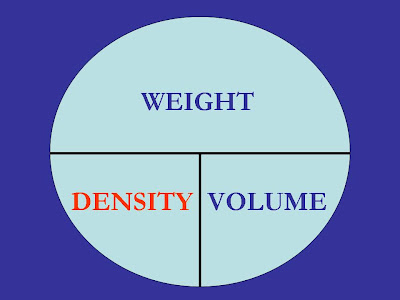# The Density EquationEverything that we have ever seen — and even things we can’t see — in the entire universe is made of “matter.” Science defines Matter as “anything that has mass and occupies space.”
On the surface of our planet, we can equate “mass” with a more familiar concept — weight. And we measure the “space” occupied by matter as volume. Today’s equation is among the most fundamental in all of science, because it compares the two basic measurements of all matter: weight and volume.
How does such a basic science formula wind up as one of the five key formulas for Water System Operators? There are several applications of this formula for us, and we’ll touch on the two most important ones in today’s blog, as we continue to prepare for the California Water Treatment Operator exam — just one week from today.
As with our other equations, we have three variables here, meaning there are three categories of questions that can be asked. On the Distribution Operator tests, we often see questions related to weight, such as “find the weight of water in this storage tank.” To solve such a problem, we would need to know the volume of water in the tank. For that, we would use the our first formula, addressed in our April 6 blog. But what is the Density of the water in this tank?
Density of any substance is dependent on that substance’s temperature. While the weight of the substance doesn’t change with temperature, its volume does. And if the volume changes, so does the density. Increasing temperature causes volume expansion; decreasing temperature causes contraction. This is true for water, too. However, over the relatively limited temperature range of a water system, we treat density as a fixed value of 8.34 pounds per gallon.
Notice the units: pounds of weight divided per gallon of volume. And while we almost always measure weight in pounds, we do use multiple units to measure volume. So another expression for density of water is 62.4 pounds per cubic foot. These are the same thing! Don’t let the units confuse you. Just use the expression that’s right for your calculation: are you using gallons or cubic feet for your volume?
In Treatment settings, we use a lot of chemicals — substances other than water. Different substances have different densities. Even when our chemicals are dissolved in water, the density is not the same as the water. For example, the density of a sodium hypochlorite solution may be 10 pounds per gallon. The actual density will depend on how concentrated the solution is: if there is just a little chemical, the density will be close to that of the water in the solution; if there is a lot of chemical, the density will normally be higher than 8.34 pounds per gallon.
And that brings us to the second important application of today’s equation. We usually measure liquids by volume, rather than by weight. But when we use the fifth of our five formulas — the “Pounds Formula” — we calculate the weight of chemical we need to apply, in pounds or pounds per day. What if we want to convert our weight answer from the Pounds Formula into a more convenient volume measurement, like gallons — as in how many gallons of bleach are needed for a disinfection operation, or how many gallons per hour should my chemical feeder be delivering? That’s when we turn to today’s formula, which allows us to switch between weight and volume easily — as long as we know the density of our chemical solution. Because there are so many possible chemicals and solution strengths, on certification tests, this has to be given to you in the problem itself — handed to you on a silver platter!